# FTCE Math: Circles & Arcs Chapter Exam

Exam Instructions:

Choose your answers to the questions and click 'Next' to see the next set of questions. You can skip questions if you would like and come back to them later with the yellow "Go To First Skipped Question" button. When you have completed the practice exam, a green submit button will appear. Click it to see your results. Good luck!

### Page 1

#### Question 1 1. In the picture below, if AC is 14 and AB is 10, what is the length of AD?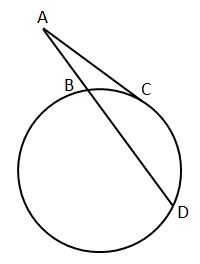#### Question 2 2. If arc AB is 72 degrees, what is the measure of angle ACB?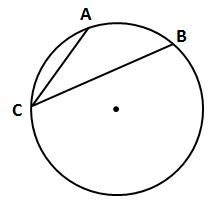#### Question 3 3. If arc AC is 106 degrees and arc BC is 82 degrees, what is the measure of angle ACB?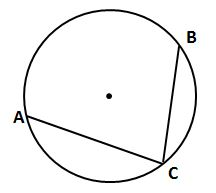### Page 2

#### Question 7 7. In the picture below, if arc CE is 172 degrees and arc BD is 80 degrees, what is the measure of angle CAE?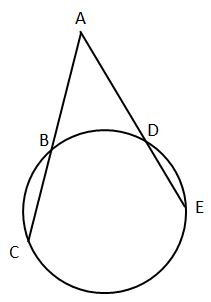### Page 3

#### Question 11 11. If angle OAB is 45 degrees, what is the measure of angle AOB?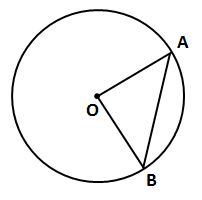#### Question 13 13. In the picture below, if AE is 10, AB is 14 and ED is 16, what is the length of CE?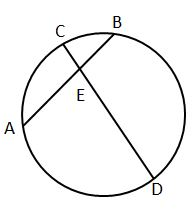#### Question 14 14. In the picture below, if AB is 7, BC is 19 and AD is 8, what is the length of DE?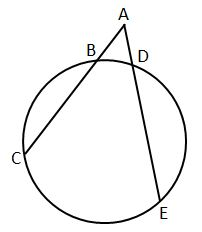### Page 4

#### Question 17 17. In the picture below, if arc BD is 168 degrees and arc BC is 70 degrees, what is the measure of angle BAD?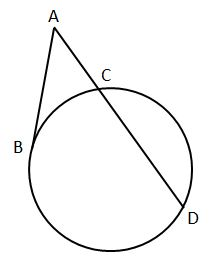#### Question 18 18. In the picture below, if AE is 8, CE is 12 and ED is 5, what is the length of EB?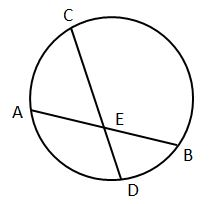#### Question 19 19. In the picture below, if arc AB is 82 degrees, what is the measure of angle BAC?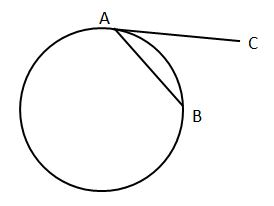### Page 5

#### Question 21 21. In the picture below, if major arc BC is 258 degrees and minor arc BC is 102 degrees, what is the measure of angle BAC?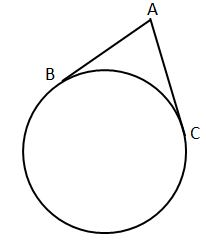#### Question 25 25. If angle ACB is 34 degrees, what is the measure of angle ADB?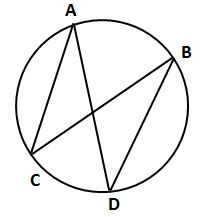### Page 6

#### Question 26 26. In the pictured circle, the measure of angle ACB is 22 degrees and the measure of angle BXD is 120 degrees. What is the measure of angle CAD?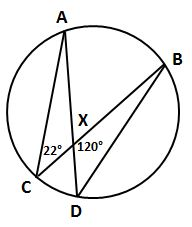#### Question 29 29. If arc BC is 84 degrees, what is the measure of angle ACB?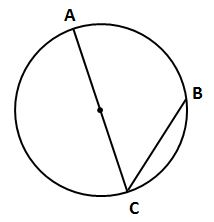#### Question 30 30. If AB and CB are tangent segments, what is the length of CB?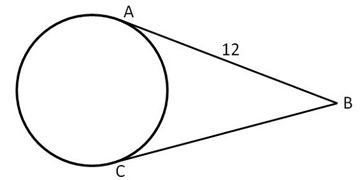#### FTCE Math: Circles & Arcs Chapter Exam Instructions

Choose your answers to the questions and click 'Next' to see the next set of questions. You can skip questions if you would like and come back to them later with the yellow "Go To First Skipped Question" button. When you have completed the practice exam, a green submit button will appear. Click it to see your results. Good luck!

Support